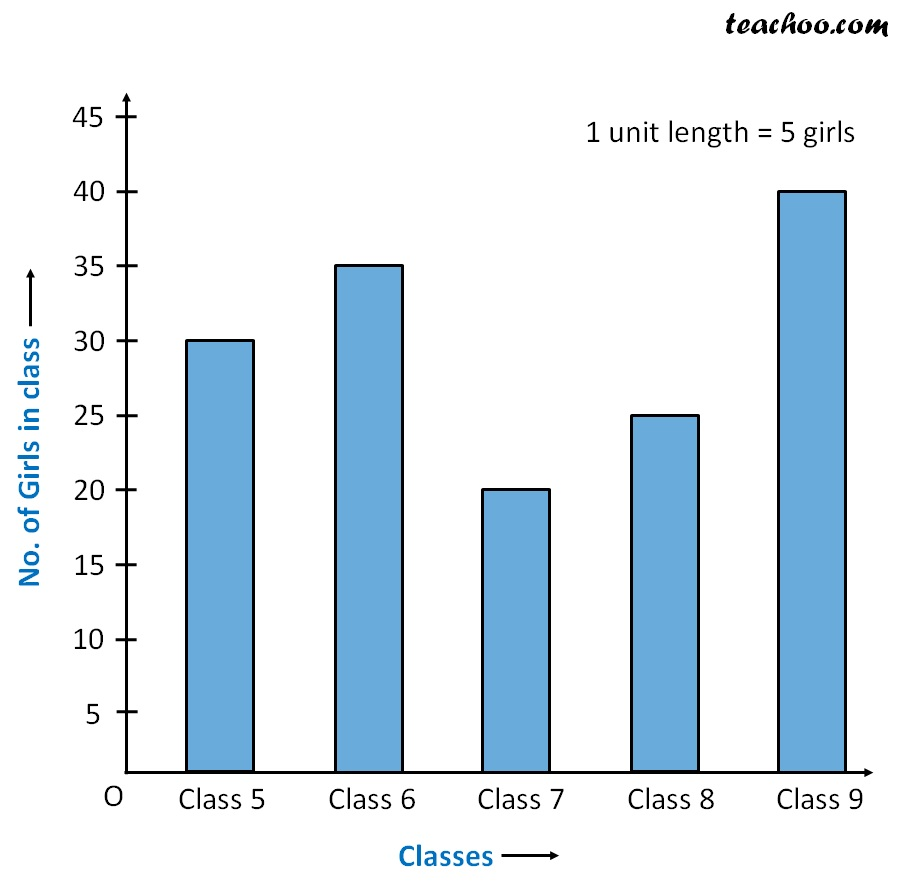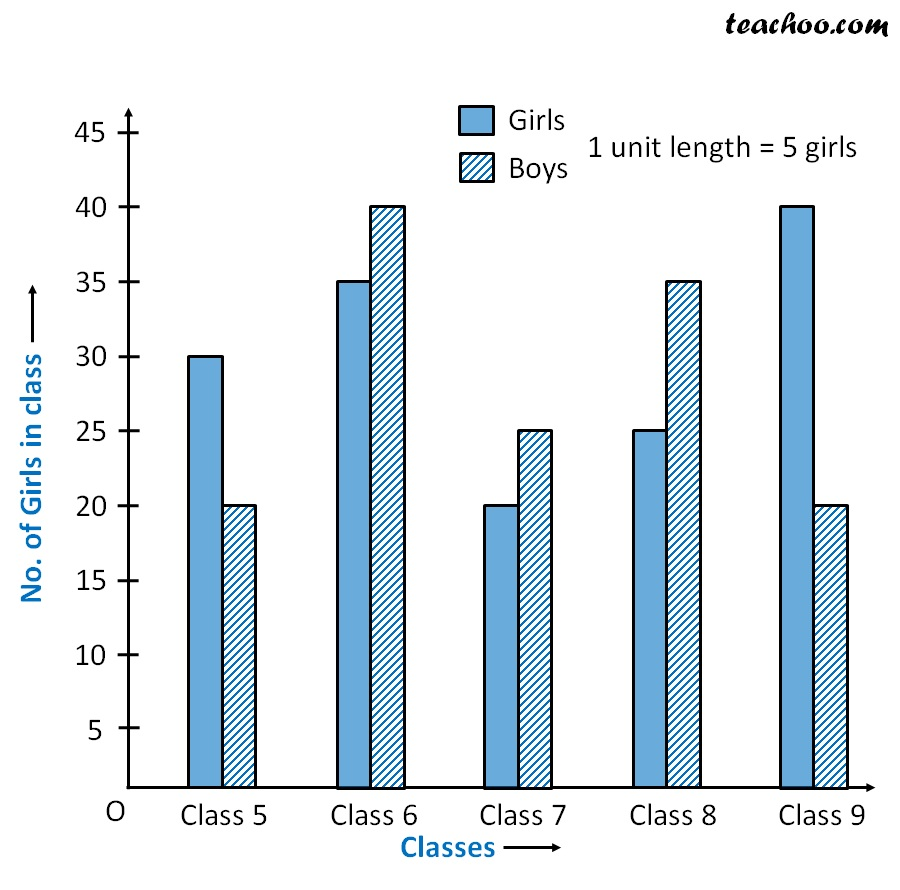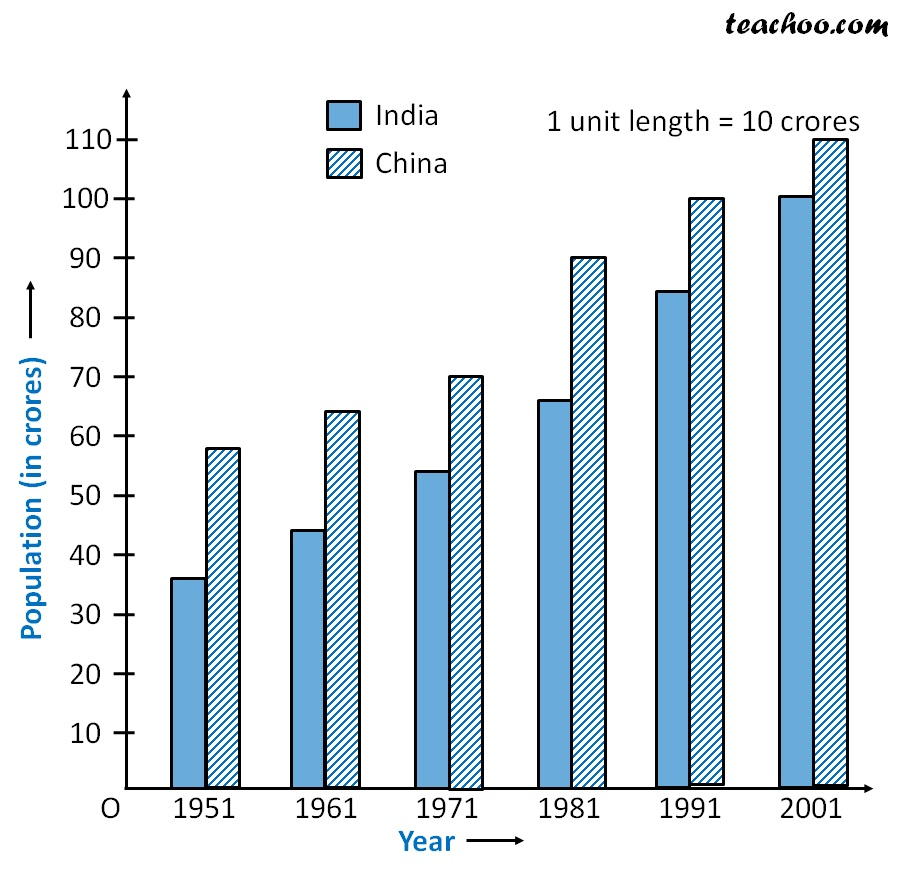Types of Graph

Chapter 13 Class 8 Introduction to Graphs
Concept wise

In Bar graph,

We made a table to a bar graph

 Class 5 th 6 th 7 th 8 th 9 th Number of girls 30 35 20 25 40But,

What if we have data like this

 Class 5 th 6 th 7 th 8 th 9 th Number of girls 30 35 20 25 40 Number of boys 20 40 25 35 20

Here, we make a double bar graph.

Since number of students are from 20 to 40

We draw bar graph from 0 to 45

with a gap of 5Let’s take another example

Suppose population of India & China are

 1951 1961 1971 1981 1991 2001 Population of India (in crores) 36 44 54 68 84 102 Population of China (in crores) 58 64 70 90 100 110

Here, population is from 36 to 110

we draw graph from 0 to 110 with gap of 10Learn in your speed, with individual attention - Teachoo Maths 1-on-1 Class

### Transcript

In Bar graph, We made a table to a bar graph But, What if we have data like this Here, we make a double bar graph. Since number of students are from 20 to 40 We draw bar graph from 0 to 45 with a gap of 5 Let’s take another example Suppose population of India & China are Here, population is from 36 to 110 we draw graph from 0 to 110 with gap of 10